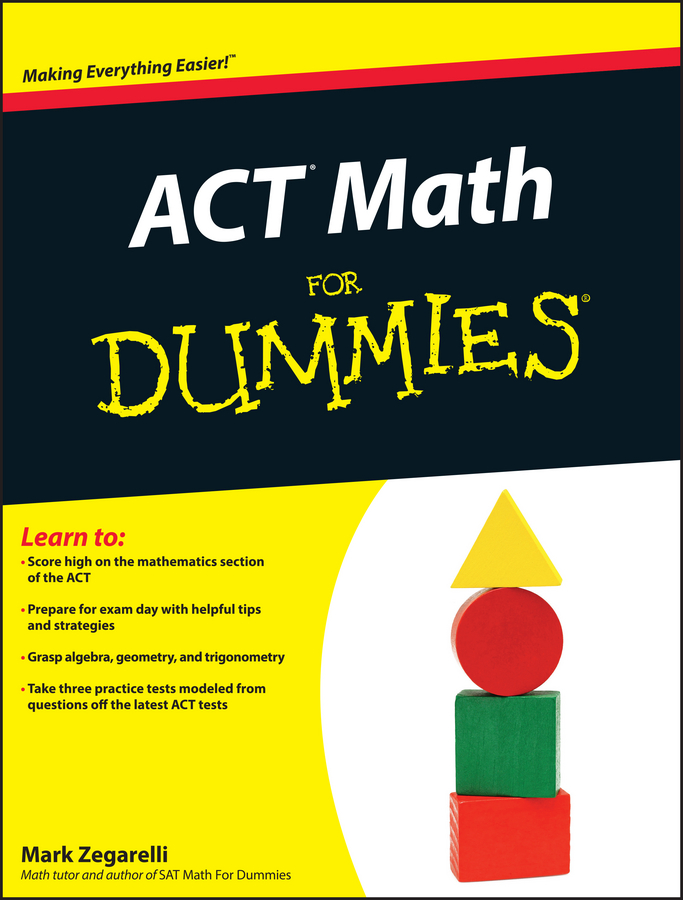##### ACT Math For DummiesThe mathematical order of operations is very important on the PSAT/NMSQT math sections. Please Excuse My Dear Aunt Sally (PEMDAS) is a mnemonic (memory aid) that helps you remember which operation comes first, which comes second, and so forth.

The order matters because if you ignore Aunt Sally, you end up with the wrong answer. And you can be sure that the testers plant wrong answers among the choices that look very appealing to anyone who forgets the proper order of operations.

You may have heard of Aunt Sally in a slightly different way, as GEMDAS. In that case think of GEMDAS as a plea to our first president: George, Excuse My Dear Aunt Sally.

Every time you see a question that requires several steps, invite Aunt Sally to the party. Here’s what each letter means, in order:

1. P is for parentheses.

Do everything in parentheses first. (If you’re working with the GEMDAS memory aid, the G stands for grouping. Whatever is in parentheses is a grouping.)

2. E stands for exponents.

Your second step is to calculate or simplify exponents (squares, cubes, and so on).

3. M means multiply, and D is divide.

Work from left to right, multiplying and dividing as you go.

Make sure you don’t multiply everything before dividing — left to right is the key!

4. A stands for add, and S means subtract.

Again, work from left to right, adding and subtracting as needed.

Some calculators have PEMDAS programmed in. Before you rely on your calculator to remember Aunt Sally, check it out. The manual that came with the calculator or the website of the manufacturer may tell you, or you can try a few sample problems to see whether PEMDAS is automatic or not. If it isn’t, enter each calculation separately.

Take a look at PEMDAS in action. Suppose you have to figure out the value of this:

551 – (220 ÷ 4 x 8) + 53

Keeping Aunt Sally in mind, begin with the Parenthesis: 220 ÷ 4 x 8. Work from left to right: 220 ÷ 4 = 55. Multiply 55 by 8 and you get 440. Now hit the Exponent. When you cube 5 (5 x 5 x 5) you get 125. Here’s what you have so far:

551 – 440 + 125

Go from left to right, and you have 551 – 440 = 111. Now you have 111 + 125, which gives you 236.

By the way, PEMDAS also works for questions in which you find a variable (a letter, such as n or x, that represents a number).

Aunt Sally loves company. Invite her to these practice problems.

1. Simplify: 1 + (2 – 4)2 + 10 +2

(A) –9
(B) –4.5
(C) 4.5
(D) 5.5
(E) 10
2. The expression 10 – 2(2 – 32) – 9 / 3 x 2 is equal to

(A) –18
(B) –9
(C) 2
(D) 18
(E) 25.5
3. Simplify –172 – (32 – 90 / 9)

(A) –181
(B) –173
(C) –172
(D) –171
(E) –163

1. E.

Parentheses first: (2 – 4) = (–2). Exponents next: (–2)2 = 4. Now you have 1 + 4 + 10 / 2. The problem has no multiplication, but you should divide the last two terms before you worry about addition or subtraction. 10 / 2 = 5, so 1 + 4 + 5 = 10, Choice (E).

2. D.

Deal with the parentheses first: (2 – 32), so you apply PEMDAS to the expression within the parentheses. Exponents first: 32 = 9, so 2 – 32 then becomes 2 – 9 = –7. Rewrite the original expression:10 – 2(–7) – 9 / 3 x 2. Now multiply and divide from left to right: 2(–7) = –14, and 9 / 3 x 2 = 3 x 2 = 6. That big nasty expression you started with now looks like 10 – (–14) – 6 = 10 + 14 – 6. Add from left to right and you end up with 18, Choice (D).

3. D.

You want to handle the parentheses first, but they include some other operations, so you need to simplify the math inside of the parentheses. In there, deal with the exponent first: 32 = 9, so you have 9 – 90 / 9. Remember that division comes before subtraction, so simplify to 9 – 10, which is –1. The whole expression is now –172 – (–1), or –172 + 1, which equals –171, Choice (D).